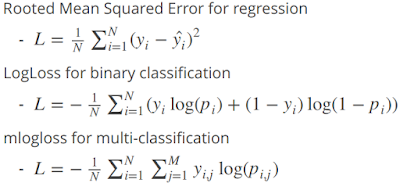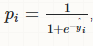# Ensemble Learning : Boosting and Bagging

In this article, we will cover the concepts of bagging and boosting. It also includes how Stochastic Gradient Boosting works.

Ensemble learning

Ensemble learning is a machine learning concept in which idea is to train multiple models (learners) to solve the same problem.

The main advantages of Ensemble learning methods are :
1. Reduced variance : Overcome overfitting problem. Low variance means model independent of training data. Results are less dependent on features of a single model and training set.
2. Reduced bias : Overcome underfitting problem. Low bias means linear regression applied to linear data, second degree polynomial applied to quadratic data. Combination of multiple classifiers may produce more reliable classification than single classifier.

Bagging (Bootstrap Aggregating)

Generates m new training data sets.  Each new training data set picks a sample of observations with replacement (bootstrap sample) from original data set.  By sampling with replacement, some observations may be repeated in each new training data set. The m models are fitted using the above m bootstrap samples and combined by averaging the output (for regression) or voting (for classification).

Example :

Random forest is one of the most important bagging ensemble learning algorithm, In random forest, approx. 2/3rd of the total training data (63.2%) is used for growing each tree. And the remaining one-third of the cases (36.8%) are left out and not used in the construction of each tree. Each tree gives a classification, and we say the tree "votes" for that class. The forest chooses the classification having the most votes over all the trees in the forest. For a binary dependent variable, the vote will be YES or NO, count up the YES votes. This is the RF score and the percent YES votes received is the predicted probability. In regression case, it is average of dependent variable.

Uses of Bagging Algorithm
1. It is designed to improve the stability and accuracy of machine learning algorithms used in statistical classification and regression.
2. It also reduces variance and helps to avoid overfitting

Boosting Algorithm

In this example, we focus on boosting algorithm, especially Adaboost algorithm.
1. Start by creating a tree on training data, where each observation is assigned an equal weight.
2. Then compute the predicted classification and weights are redetermined and assign greater weight to those observations that are difficult to classify and lower weights to those that are easy to classify. Weights for all observations must sum to 1.
3. Second tree is grown on weighted data (weights are based on residuals or misclassification error). Idea is to improve prediction of first tree.
4. Weights are redetermined and assign higher weights if it is classified incorrectly.
5. New Model is now Tree 1 + Tree 2
6. Compute residuals or classification error from this new 2-tree model (Tree1 + Tree2) and grow 3rd tree to predict revised residuals.
7. Then subsequent trees help in classifying observations that are not well classified by preceding trees.
8. The final prediction is a weighted sum of the predictions made by previous tree models.
In this process, we have created many simple decision trees, where each tree is built for the prediction errors (classification error) of the previous tree.

Why Boosting works?

We weight misclassified observations in such a way that they get properly classified in future iterations.

Main Idea
• Combine several simple decision trees (can be hundred or thousands)
• Each tree complements the previous ones (Avoid redundancy)
• Keep track of the errors of the previous trees
This technique is faced with a problem of overfitting

To overcome problem of overfitting, stochastic gradient boosting comes into picture.

A general solution to the overfitting problem is to evaluate the quality of the fitted model by predicting observations in a test-sample of data that have not been used before to estimate the respective model(s). In Stochastic Gradient Boosting (GBM), a similar solution is implemented.

It tries to optimize the loss function by tuning different values of weights or coefficients to minimize the error. It is a method which comprises a vector of weights (or coefficients) where we calculate their partial derivative with respective to zero. The motive behind calculating their partial derivative is to find the local minima of the loss function (Residual Sum of Squares), which is convex in nature.

To train the model, we need to optimize a loss function. It is the function we need to minimize.Loss Functions

Loss or Error Function for Classification

Minimizing Loss Function means maximising accuracy of the classifier.
Gradient Boosting minimize logistic regression's LogLoss (aka negative log-likelihood or bernoulli function)Bernoulli distribution is a special case of the binomial distribution with n=1. AdaBoost minimizes Exponential Loss function.

Log Loss or Bernoulli :

LogLoss can be expressed in form of
Logloss = [∑ y log(pᵢ) + (1-y) log(1 - pᵢ)] * -(1/N)
Here, Pi is logistic function. Refer the image below -logistic function

LogLoss = -(sum((actual*log(predicted)+(1-actual)*log(1-predicted)) / length(actual)
In this case, actual means 1 and predicted means predicted probability (It's same probability that is derived from model).

The idea is to minimize the negative log-likelihood. It can also be read as maximizing log-likelihood.
The reason for taking the negative of the logarithm of the likelihood are as follows -It is because log(probability) will be negative so we take negative of log(p) to make it positive so that it would easy for comparison.

Loss function for Regression : Squared Error
(y - f(x))^2
It is called squared-loss function. y-f(x) : residual

Important Points :
1. Residuals can be interpreted as negative gradients.
2. For Classification, Gradient Descent Loss Function = Log-loss function.
3. For Regression, Gradient Descent Loss Function = Squared loss function.

1. Simple tree is built on original target variable by taking only a randomly selected subsample of the dataset. It implements 'Stochastic' boosting techniqueParameter :  bag.fraction :  sampling rate (0.5). In this case, 0.5 implies only 50% of the data used at each iteration.
2. The tree is built as small as two nodes and typically in the range of 4-8 nodes (Parameter:  interaction.depth : Nodes per tree)
3. We score full training data using this tree model and compute the negative gradient (i.e. residual or classification error) for every record
4. Make residual as a new target (dependent) variable
5. Grow second tree to predict the residuals from first tree. Idea is to improve first tree.
6. Each tree is weighted by a small weight prior to being used to compute the current prediction or score produced by the model.This means that the model prediction changes by very small amounts after a new tree is added to the model. It is to reduce the impact of each additional tree. The weighting factor is also called shrinkage or learning rate.
7. We combine two trees by taking score for any record that come from the first tree and add to it the score that comes from the second tree. The second tree can therefore be thought of as correcting the predictions of the first tree. So a model from the end of a Gradient Boosting process is simply the sum of all the scores of all the trees that were built.
8. Now, we have revised model with two trees. Third tree is grown on residuals from model consisting of first two tree. The whole process keeps on going.
9. It yield models (i.e. additive weighted expansions of simple trees) that generalize well to new observations, i.e., exhibit good predictive validity.
Also, like in bagging, subsampling allows one to define an out-of-bag estimate of the prediction performance improvement by evaluating predictions on those observations which were not used in the building of the next base learner. Out-of-bag estimates help avoid the need for an independent validation dataset, but often underestimate actual performance improvement and the optimal number of iterations.

Important Point
Prediction in Gradient Boosting models are made not by combining voting of all the trees, but cumulative predictions of all the trees.

Difference between Bagging and Boosting
1. In Boosting, each model is built on top of the previous ones. Whereas in bagging each model is built independently.
2. The final boosting ensemble uses weighted majority vote while bagging uses a simple majority vote.
3. Bagging is a method of reducing variance while boosting can reduce the variance and bias of the base classifier
4. Boosting is better than bagging on non-noisy data
5. Bagging is effective more often than boosting

R Packages for Boosting and Bagging
1. gbm - The gbm package offers two versions of boosting for classification (gentle boost under logistic and exponential loss). In addition, it includes squared error, absolute error, Poisson and Cox type loss functions.
2. ada - Performs discrete, real, and gentle boost under both exponential and logistic loss on a given data set.
3. randomForest - Random Forest Package (Bagging)
Related Posts
ShareDeepanshu founded ListenData with a simple objective - Make analytics easy to understand and follow. He has over 10 years of experience in data science. During his tenure, he worked with global clients in various domains like Banking, Insurance, Private Equity, Telecom and HR.

2 Responses to "Ensemble Learning : Boosting and Bagging"
1.Prior to installing any manual boost controller, it is important to have already added a high flowing exhaust and an aftermarket air intake/ air filter. This will ensure the car is able to breath, and will allow the turbo to spool easier.
increase web traffic

2.What will be the response variable value in the 2nd model of GBM for classification problem?

Next → ← Prev